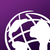# python script not working

848
4
10-17-2018 08:08 AMNew Contributor

I am trying to find out why the python script that I wrote isnt working. I am trying to count  the number of stairs in a stairwell. I have two shapefiles and i wrote the script to run auto and process how many stairs there are.  if someone can help me with this I would appreciate it thanks.

Tags (2)
4 RepliesbyMVP Esteemed Contributor

Sharing with Python‌ since this looks to be ArcPy and not ArcGIS API for Python.

Please paste the code (not a screenshot) and use /blogs/dan_patterson/2016/08/14/script-formatting?sr=search&searchId=bdf45239-640f-4c59-b3e5-bbc4c8d...‌.  Also, provide the error and traceback if you are getting an error.  If you are seeing unexpected results, describe what you are seeing and what you expect.New Contributor

here is the code below. again im not too  familar with  python  so  i am asking for some help

def createInternalParallelLines():
# import libraries
import arcpy

# set input/output parameters
polyFC = arcpy.GetParameterAsText(0) # input polygons
outParallel = arcpy.GetParameterAsText(1) # output parallel lines
lineSpacing = arcpy.GetParameterAsText(2) # line spacing
buffDist = arcpy.GetParameterAsText(3) # inner buffer distance

# parse numbers from parameters
lineSpaceNum = float(lineSpacing.split(' '))
buffNum = float(buffDist.split(' '))

# establish spatial reference
desc = arcpy.Describe(polyFC)
SR = desc.spatialReference

# set overwrite environment
arcpy.env.overwriteOutput = True
arcpy.env.outputCoordinateSystem = SR

parallels = []
# create hull rectangle to establish a rotated area of interest
coordSplit = row.hullRectangle.split(' ')

# collect corner coordinates
coordList = arcpy.Array([arcpy.Point(coordSplit,coordSplit),arcpy.Point(coordSplit,coordSplit),arcpy.Point(coordSplit,coordSplit),arcpy.Point(coordSplit,coordSplit),arcpy.Point(coordSplit,coordSplit)])

# create lines from hull rectangle
currentLines = []
for pointNum in range(0,4):
arcpy.Array([coordList.getObject(pointNum),coordList.getObject(pointNum+1)])
hullRecLine = arcpy.Polyline(arcpy.A
# loop through each input shape
for row in arcpy.da.SearchCursor(polyFC, ["SHAPE@"], spatial_reference=SR):

# create inner buffer
polyBuff = row.buffer(buffNum * -1)
rray([coordList.getObject(pointNum),coordList.getObject(pointNum+1)]))
currentLines.append(hullRecLine)

# compare first and second line to determine if first line is short or long
firstLong = 0
if currentLines.length < currentLines.length:
firstLong = 1

# calculate number of points needed along short axis
numPoints = int(math.floor(currentLines[firstLong].length/lineSpaceNum))

# create and join points to create parallel lines
for point in range(1,numPoints+1):
shortPoint1 = currentLines[firstLong].positionAlongLine(lineSpaceNum*point)
shortPoint2 = currentLines[firstLong + 2].positionAlongLine(currentLines[firstLong + 2].length - (lineSpaceNum*point))
parallel = arcpy.Polyline(arcpy.Array([shortPoint1.centroid,shortPoint2.centroid]), SR)

# intersect parallel lines with buffer
parallelBuff = parallel.intersect(polyBuff,2)
parallels.append(parallelBuff)

# write geometries to disk
arcpy.CopyFeatures_management(parallels, outParallel)

mxd = arcpy.mapping.MapDocument("CURRENT")
dataFrame = arcpy.mapping.ListDataFrames(mxd, "*")

del rowNew Contributor

Here is the code… thanks for your help

def createInternalParallelLines():

1. import libraries

import arcpy

1. set input/output parameters

polyFC = arcpy.GetParameterAsText(0) # input polygons

outParallel = arcpy.GetParameterAsText(1) # output parallel lines

lineSpacing = arcpy.GetParameterAsText(2) # line spacing

buffDist = arcpy.GetParameterAsText(3) # inner buffer distance

1. parse numbers from parameters

lineSpaceNum = float(lineSpacing.split(' '))

buffNum = float(buffDist.split(' '))

1. establish spatial reference

desc = arcpy.Describe(polyFC)

SR = desc.spatialReference

1. set overwrite environment

arcpy.env.overwriteOutput = True

arcpy.env.outputCoordinateSystem = SR

parallels = []

1. create hull rectangle to establish a rotated area of interest

coordSplit = row.hullRectangle.split(' ')

1. collect corner coordinates

coordList = arcpy.Array([arcpy.Point(coordSplit,coordSplit),arcpy.Point(coordSplit,coordSplit),arcpy.Point(coordSplit,coordSplit),arcpy.Point(coordSplit,coordSplit),arcpy.Point(coordSplit,coordSplit)])

1. create lines from hull rectangle

currentLines = []

for pointNum in range(0,4):

arcpy.Array()

hullRecLine = arcpy.Polyline(arcpy.A

1. loop through each input shape

for row in arcpy.da.SearchCursor(polyFC, ["SHAPE@"], spatial_reference=SR):

1. create inner buffer

polyBuff = row.buffer(buffNum * -1)

rray())

currentLines.append(hullRecLine)

1. compare first and second line to determine if first line is short or long

firstLong = 0

if currentLines.length < currentLines.length:

firstLong = 1

1. calculate number of points needed along short axis

numPoints = int(math.floor(currentLines[firstLong].length/lineSpaceNum))

1. create and join points to create parallel lines

for point in range(1,numPoints+1):

shortPoint1 = currentLines[firstLong].positionAlongLine(lineSpaceNum*point)

shortPoint2 = currentLines[firstLong + 2].positionAlongLine(currentLines[firstLong + 2].length - (lineSpaceNum*point))

parallel = arcpy.Polyline(arcpy.Array(), SR)

1. intersect parallel lines with buffer

parallelBuff = parallel.intersect(polyBuff,2)

parallels.append(parallelBuff)

1. write geometries to disk

arcpy.CopyFeatures_management(parallels, outParallel)

mxd = arcpy.mapping.MapDocument("CURRENT")

dataFrame = arcpy.mapping.ListDataFrames(mxd, "*")

del rowbyMVP Legendary Contributor